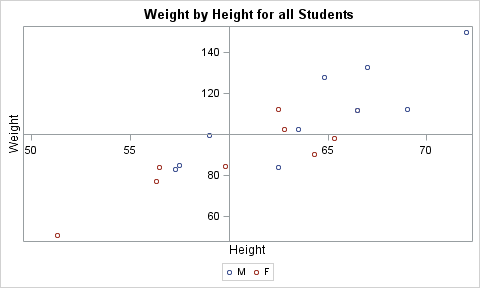## SGPlot axes through origin with negative data

I'm creating a scatterplot using sgplot. My points have both positive and negative values. I would like to draw two axes through (0,0), but keep the axis labels at the edge of the graph. What's the best way to do this? (I know I can use lineparm, but I'm hoping there is a simpler option).

1 ACCEPTED SOLUTION

Accepted Solutions

## Re: SGPlot axes through origin with negative data

REFLINE 0 / axis=x;

REFLINE 0 / axis=y;

That draws the reference lines, but leaves the axes automatically positioned.

5 REPLIES 5

## Re: SGPlot axes through origin with negative data

REFLINE 0 / axis=x;

REFLINE 0 / axis=y;

That draws the reference lines, but leaves the axes automatically positioned.

## Re: SGPlot axes through origin with negative data

Many thanks. That solves my current problem. For future reference (if I want to present this data differently) how do I get the axes with tickmarks and labels to go through (0,0)?

## Re: SGPlot axes through origin with negative data

I'll let someone else advice as to how it might be accomplished.  In my opinion, you shouldn't do it. The center of the plot should be reserved for the data, with the axis ticks, values, and labels positioned to the outside. This is based on basic principles of graphing (a la Tufte) which state that the data should be presented as purely and unadorned as possible.

## Re: SGPlot axes through origin with negative data

Another thought: To obtain a whole grid of reference lines, where each line is aligned with axes ticks, use the GRID option on the XAXIS and YAXIS statements, like this:

``````xaxis grid;
yaxis grid;
``````

That gives you all of the advantages of axes that cut through the plot area, but keeps the tick values and labels on the outside of the plot area.Jay54
Meteorite | Level 14

## Re: SGPlot axes through origin with negative data

I don't think there is a way to do this in SGPLOT.  However, you can do this using GTL.

In your case, your data would span across (0, 0), and you would set the x and y origins at zero.proc template;
define statgraph class;
begingraph;
entrytitle 'Weight by Height for all Students';
layout overlay / xaxisopts=(linearopts=(origin=60))
yaxisopts=(linearopts=(origin=100));
scatterplot x=height y=weight / group=sex name='a';
discretelegend 'a';
endlayout;
endgraph;
end;
run;

proc sgrender data=sashelp.class template=class;
run;

Discussion stats
• 5 replies
• 2113 views
• 1 like
• 3 in conversation